# Measuring

### In English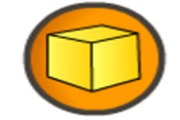### Bildetema

Learn the names of the units of measurement, weight and geometry in Norwegian and English.

### In Norwegian### Målemesteren

Practise various units of measurement for weight, volume, time and money.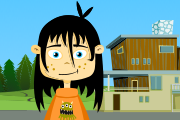### Salaby

Mathematics 1–2 Length and area. Various exercises and games.### Salaby

Mathematics 1–2 Time and clocks. Various exercises and games.### Dagen i dag

Learn dates and times.### Matematikk 5-7

On Elevkanalen’s mathematics page, you can watch videos and learn about numbers, equations, geometry, measurements, statistics and probability.### MatteMestern

Learn mathematics by watching, listening and doing exercises in Norwegian.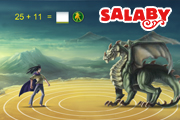### Salaby

Learn about weights and measurements in mathematics through fun exercises and games. Choose a topic. Choose between Bokmål and Nynorsk. Includes support in several languages.### Salaby

Learn about weights and measures through fun exercises and games.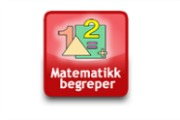### Tema morsmål

Illustrated list of mathematical terms in English and Norwegian (bokmål).### Tema morsmål

Illustrated list of mathematical terms in English and Norwegian (nynorsk).### Dagen i dag

Learn dates and times.### FBU

Get an overview of words and concepts from maths in Norwegian and in your language, with illustrations.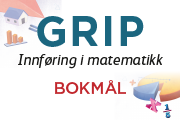### GRIP

Book with texts and exercises in counting, addition, subtraction, multiplication, division, positive and negative numbers, fractions, percentages, equations.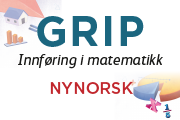### GRIP

Book with texts and exercises in counting, addition, subtraction, multiplication, division, positive and negative numbers, fractions, percentages, equations.### Jeg fikser matte

Mathematics for year 8-10. Overviews and explanations with mother-tongue support.### Kikora

Matteoppgaver innen temaene målestokk og formlikhet.### MatteMestern

Learn mathematics by watching, listening and doing exercises in Norwegian.### Tema morsmål

Illustrated list of mathematical terms in English and Norwegian (bokmål).### Tema morsmål

Illustrated list of mathematical terms in English and Norwegian (nynorsk).### Tospråklig matematikk 8-10

On Elevkanalen’s bilingual mathematics page, you can watch lots of videos. You can learn about numbers, fractions and percentages, algebra, equations and formulas, measurements, geometry, graphs and functions, statistics, probability and economics. In the menu, you can choose between Norwegian (Bokmål), Arabic, Dari, Pashto, Kurmanji and Tigrinya.